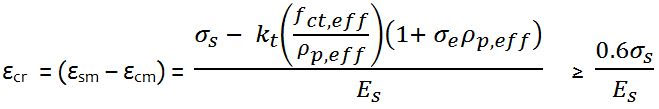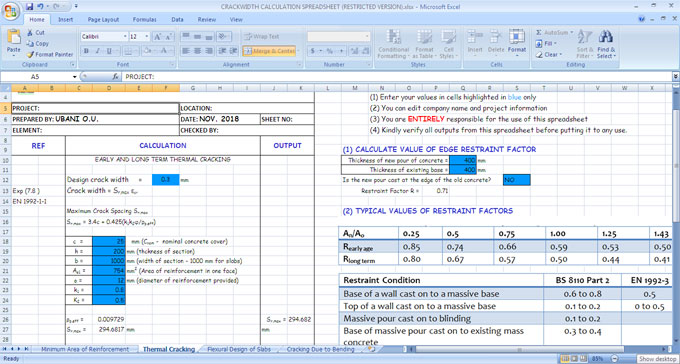# How to measure crackwidth caused by bendingCracking in reinforced concrete structures normally occurs because of various factors like bending, shear, twisting, axial tension, and restraint from movement. As the tensile strength of concrete is weak, so the cracking is inevitable. Cracking normally stands for a serviceability limit state problem. Besides, damaging concrete, the cracking also leads to stability issues, and leakage problem in water retaining structures.

Cracking is supposed to take place in a concrete section when the restraint strain surpasses the tensile strength ability of the concrete. It signifies that when cracking takes place, some part or the entire of the concrete section should be in tension. Crackwidth in concrete is estimated by multiplying crack inducing strain (strain dispersed by the incidence of cracking) with the crack spacing.

The strain that produce crack because of flexure (bending) is demonstrated in expression 7.9 of EN 1992-1-1 as;where;
= denote strain in reinforcement.
= denote strain in the concrete among cracks.
= stress in the reinforcement depending on cracked section properties under quasi permanent load combination
ae = modular ratio, Es/Ecm (normally a value of 7 may be used.)
fct,eff = fctm at 3 days and/or 28 days.
?p,eff = As/Ac,eff (this is computed for each face)

Where; As = area of reinforcement provided, mm2
Ac,eff = Area of concrete in tension whose depth is:
min[0.5h , 2.5(c + 0.5?) , (h ? x)/3] for each face of a wall

where;
h = thickness of wall
c = nominal cover
? = bar diameter
x = depth to neutral axis
d = effective depth.
Es = elastic modulus for reinforcement = 200,000 MPa

Solved Example
Workout the crackwidth because of externally applied load on a 200 mm thick slab with the following data;
Design serviceability bending moment = 14.96 kNm/m
Area of tension reinforcement provided at ultimate limit state As1 = H12@200 c/c (Asprov = 565 mm2/m)
Concrete cover = 25 mm
Effective depth d = 200 - 12/2 - 25 = 169 mm